## Global Temperature Forecast Using Prophet and CO2

In this article I will leverage the global temperate dataset I discussed previously to make a temperature forecast using Facebook Prophet for the next 50 years. Note: the temperature dataset serves ONLY as a vehicle to learn how to do forecasting using Prophet. In general, climate and other complex sciences cannot be solved using a simple tool such ash Prophet.

All code can be found in this gist.

#### Data

To review, the temperature dataset covers monthly data since 1850 including 95% confidence intervals (high CI - blue, low CI - red):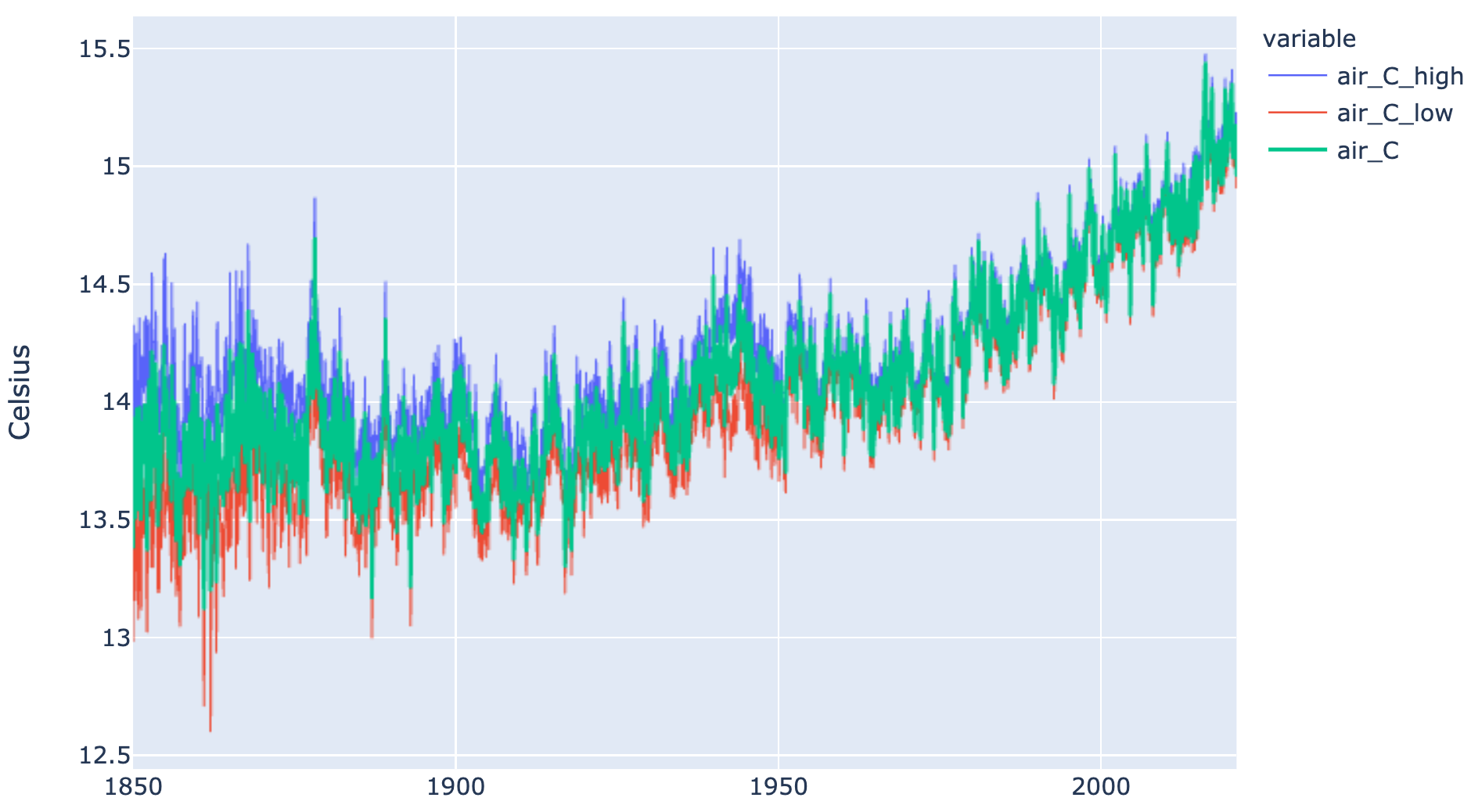In addition, I will use the CO2 emmissions data from ourworldindata.org: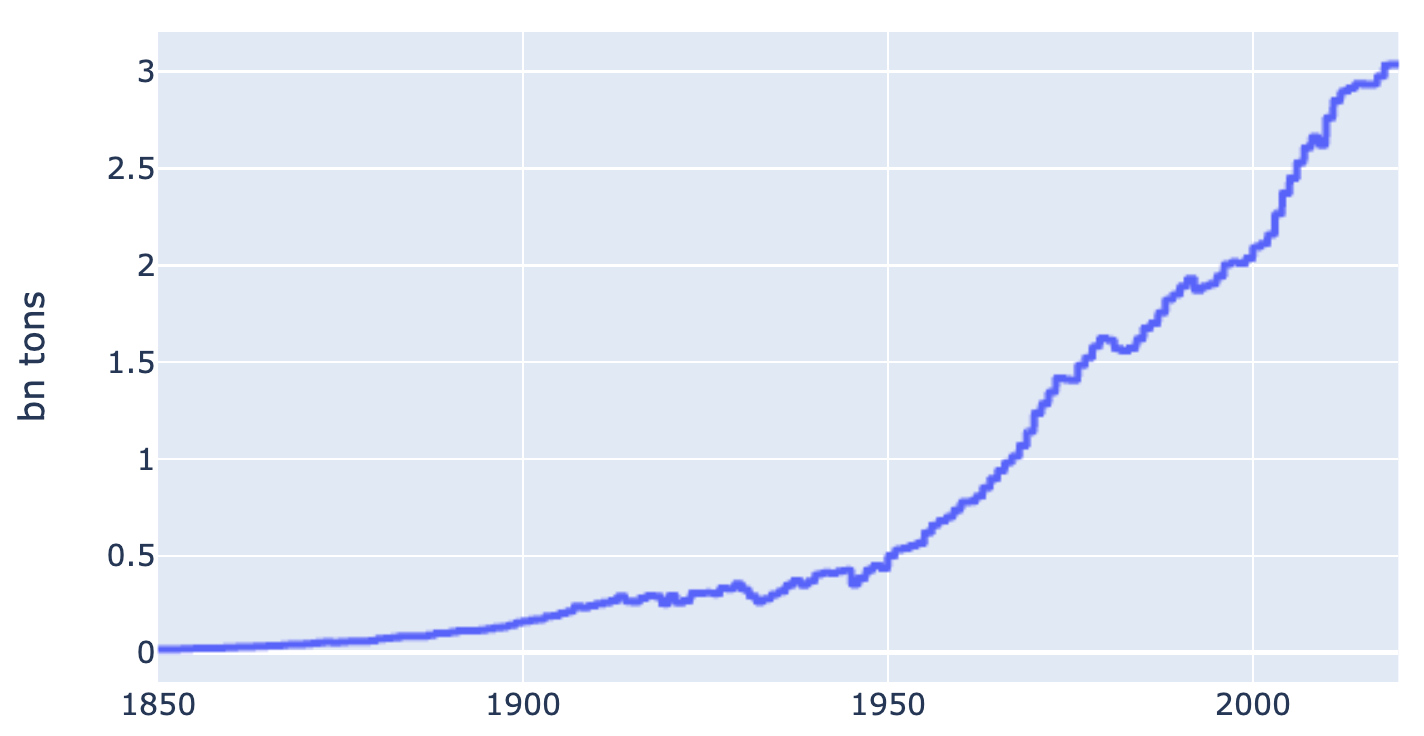#### Forecast

I will only highlight here how the Prophet API works (specifically when we want include an additional regressor such as CO2). First, we need to format the training dataset such that the label column is y and date is ds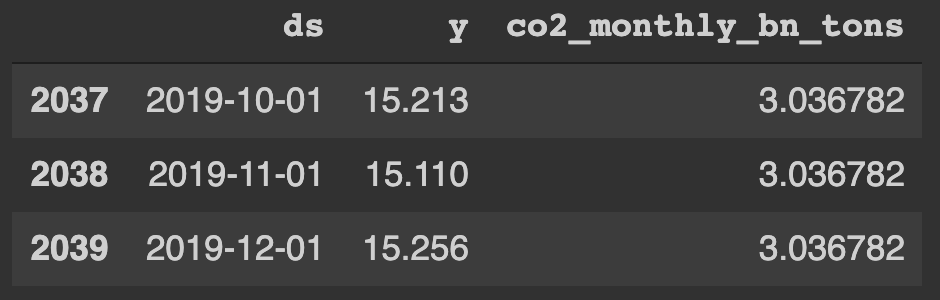Next, we train the Prophet model and add the custom regressor (CO2):

```m = Prophet()
prior_scale=0.5,
mode="multiplicative",
standardize=True)
m.fit(prophet_train_set)
```

Then we need to create a forecast dataset that includes the dates to be forecasted and assumptions for the custom regressor. In the temperature forecasting dataset, I created timestamps for the next 50 years. Last 3 rows of the forecast dataset ("prophet_forecast_set"):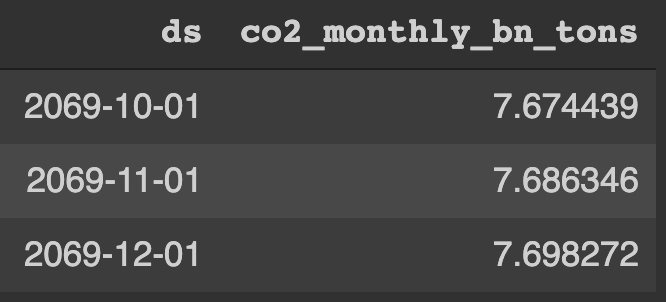In order to create the dataset above, I had to make an assumption about CO2 growth. I assumed that monthly growth over the next 50 years will continue at the same pace as it has between 2000-2020: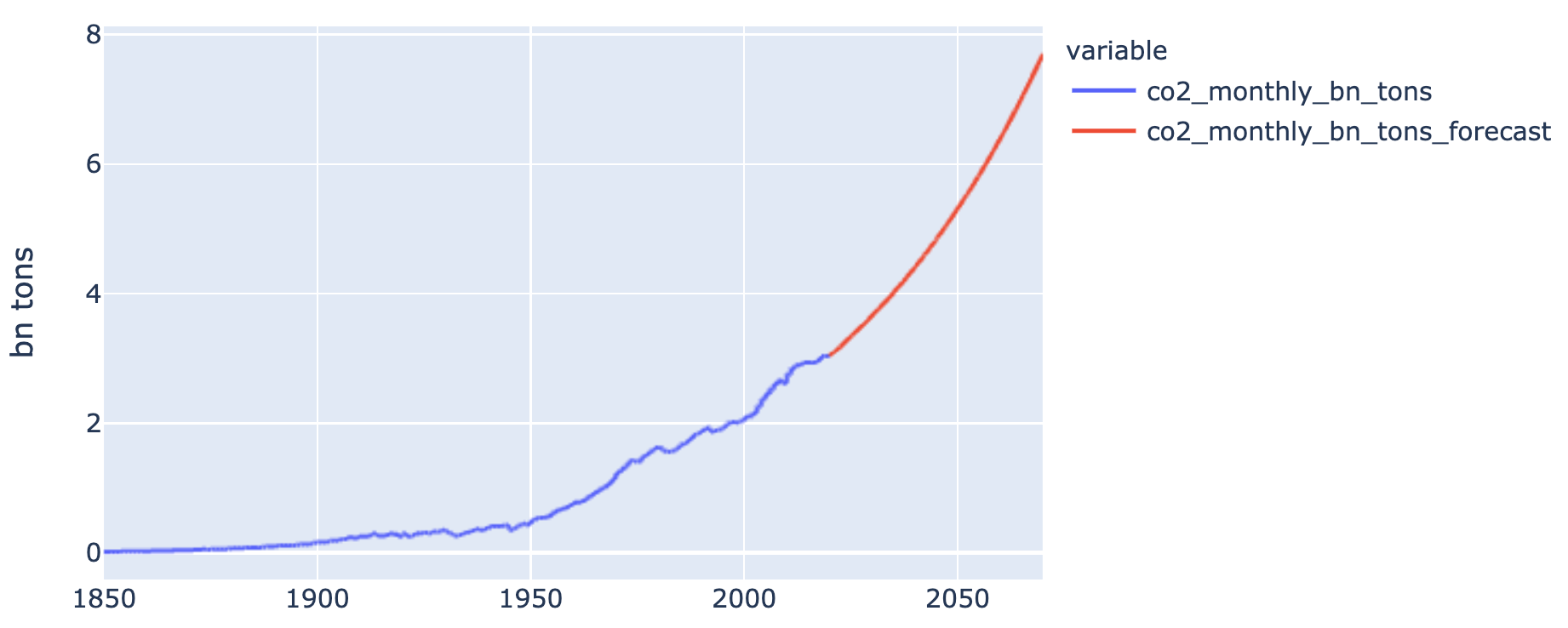In reality, the value of the temperature forecast comes from the data scientist's background knowledge of the field. In this example, in order for the temperature forecast to be valuable, we have to be able to forecast CO2 emissions (and other regressors) with high confidence.

Performing the actual forecast using Prophet is very simple:

```forecast_prophet = m.predict(prophet_forecast_set)
```

Prophet generates valuable confidence intervals for its forecast. These confidence bars are more valuable than the point forecast itself. In the chart below, the point forecast in 2070 is 16.1C. However, the forecast ranges widely from nearly 17C to 15.2C.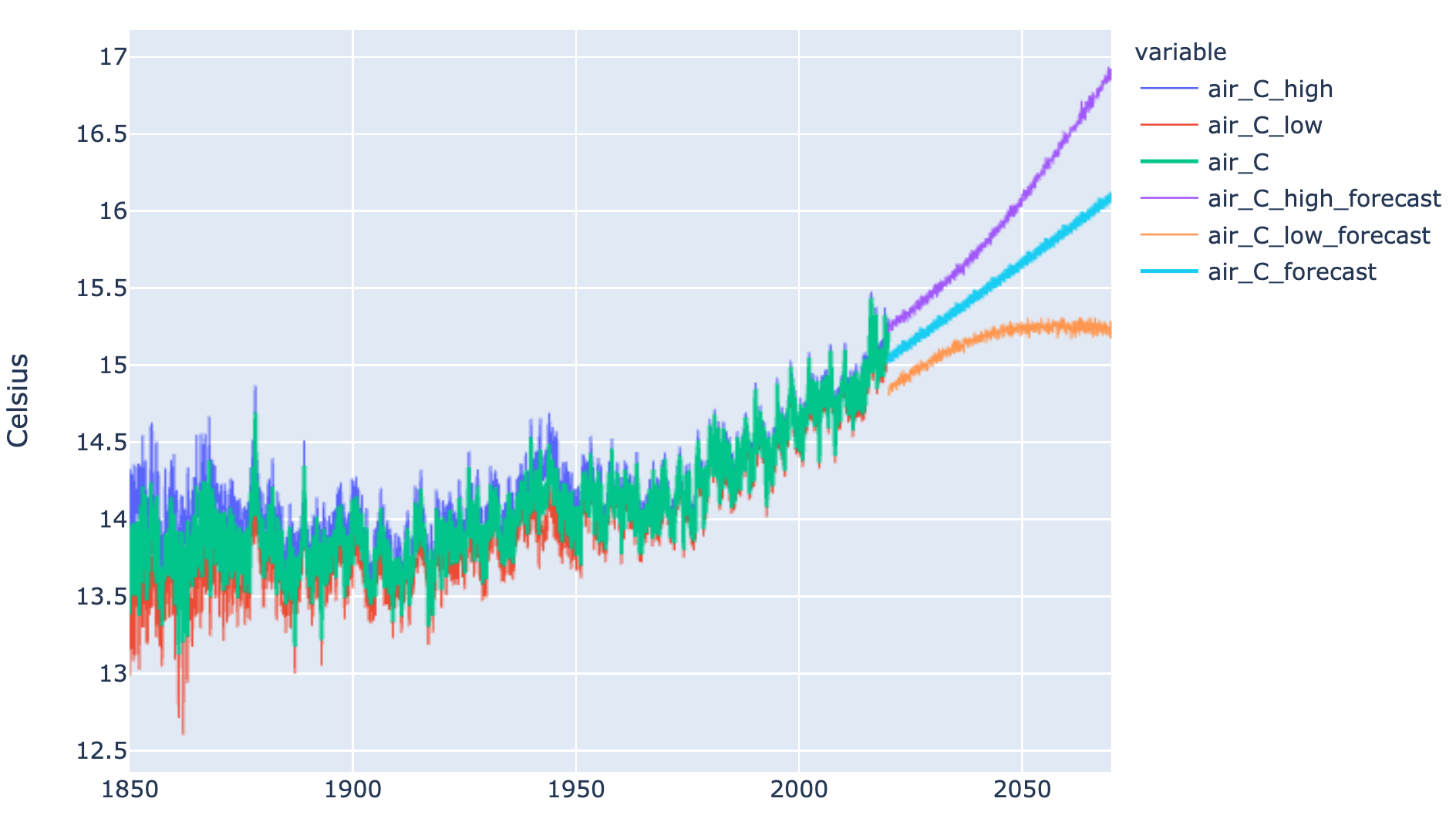#### Validation

The step that many people doing forecasts "conveniently" skip is validation. In other words, if we approached the problem the same way in the past, how incorrect would we turn out to be today.

Let's assume that we are standing in 1970, and we apply the exact same methodology as above to forecast the next 50 years (so we are forecasting 1970-2020). What would the forecasting graphs look like compared to the reality we've already experienced? First, our hypothetical CO2 assumption would match reality reasonably nicely: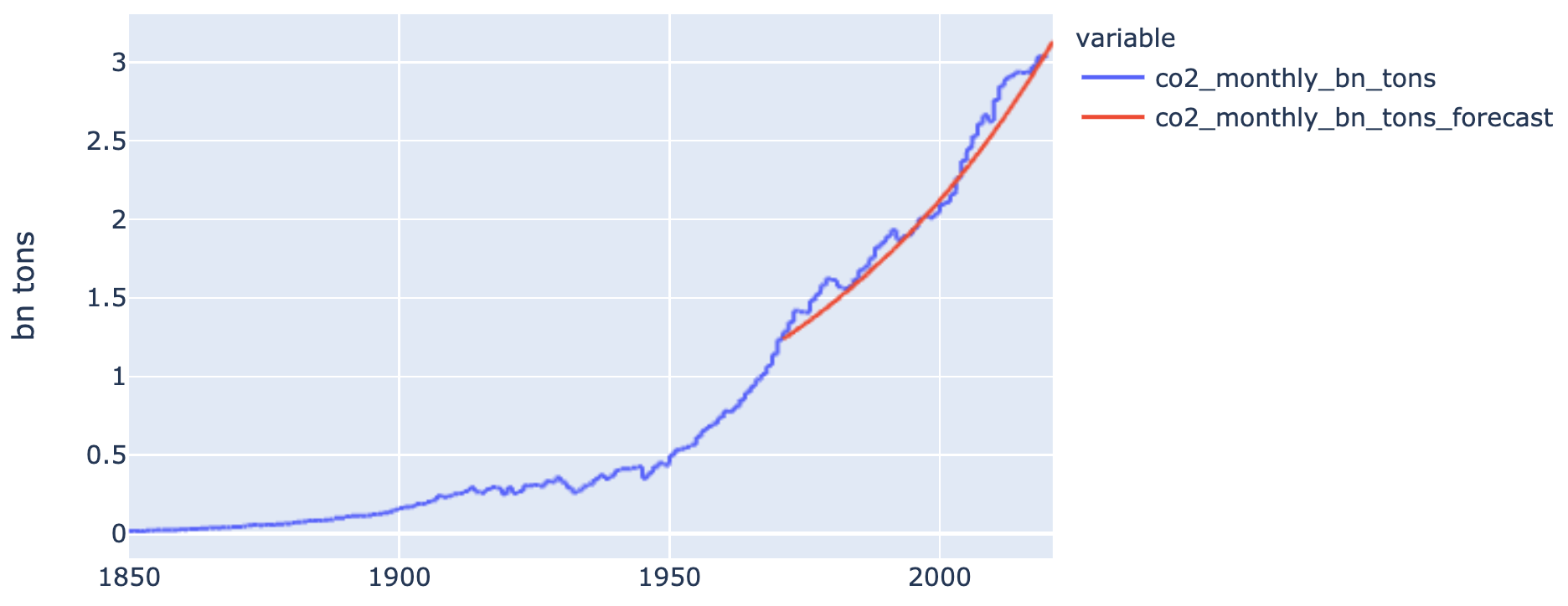However, our temperature point forecast would underestimate reality. Our forecast is still within confidence intervals because it nearly perfectly aligns with the upper bound. However, the behavior of the forecast doesn't appear to reflect the upward slope we've experienced historically: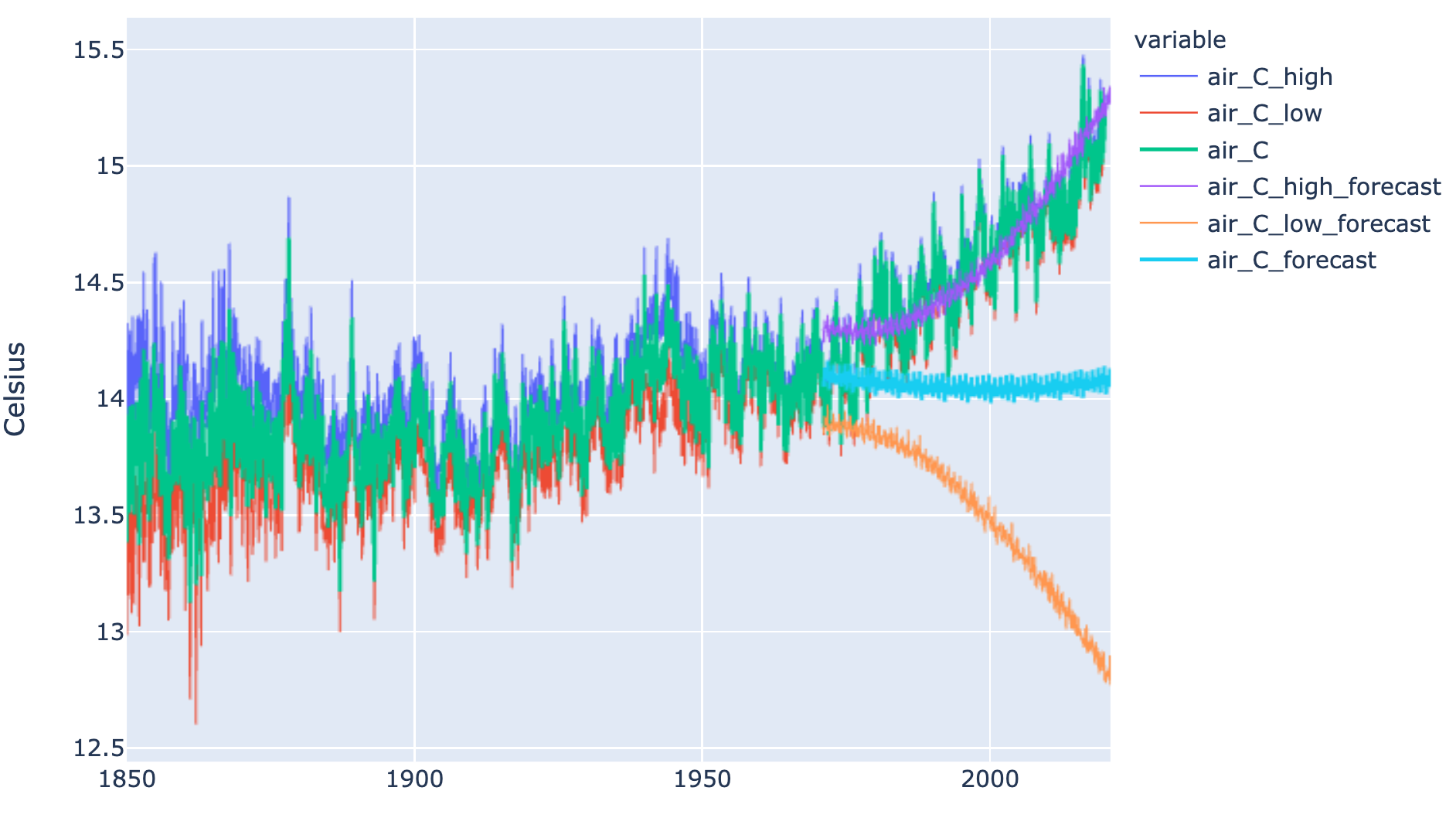This is an example of why confidence intervals are more important than point estimates. Also, it reflects how important it is to be intellectually honest when forecasting and performing historical validation. The takeaway here might be that we are missing additional regressors to be able to properly forecast the temperature physical process.